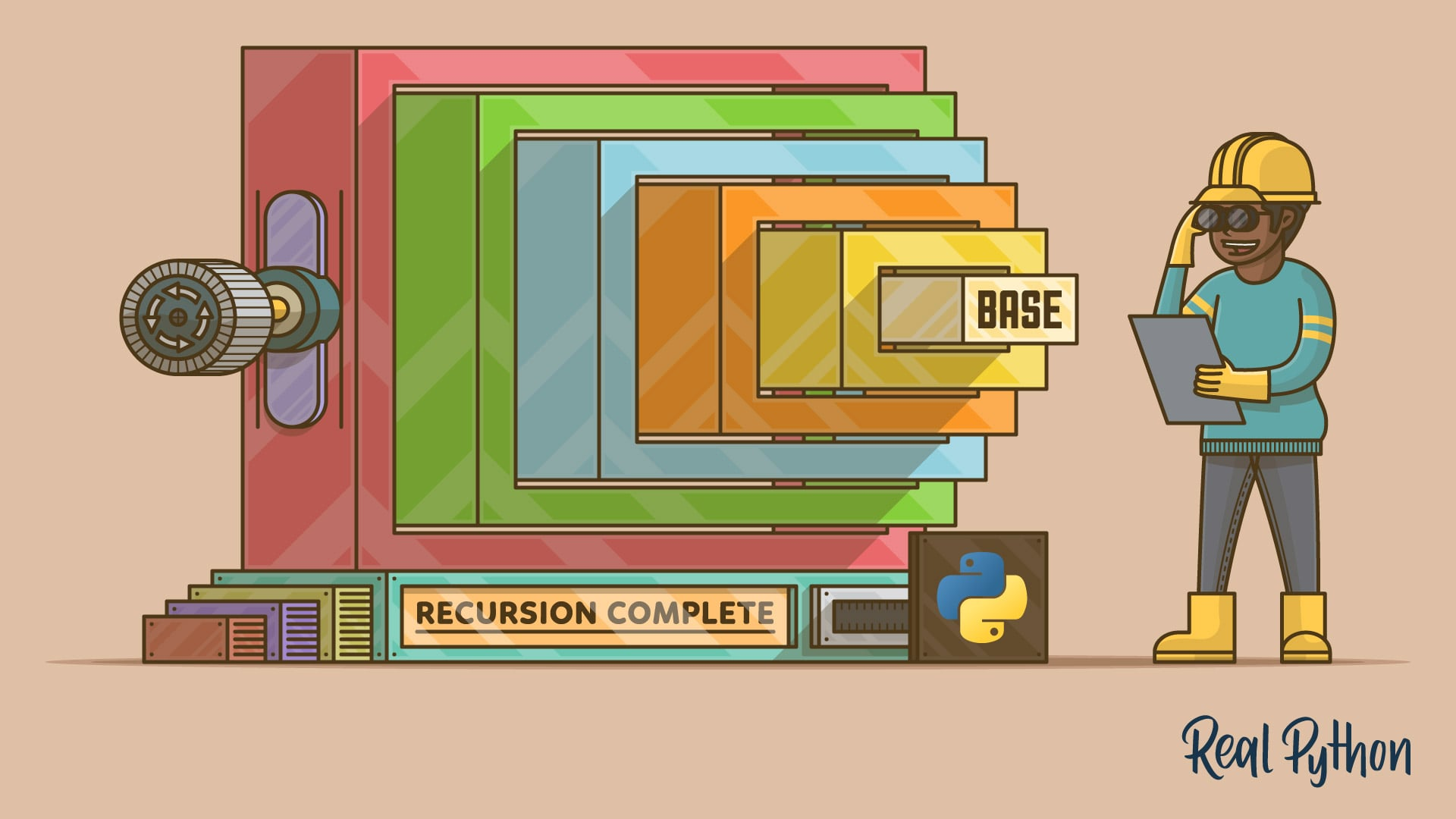# Acquired Taste for Recursion Part 3- Recursion, QuickSort, & Partition

Posted by Samuel.Ogundiran on April 15, 2021Everything we have worked for brought us to this point. If you have not seen my previous article leading up to this post, go check them out here.

articule 1

articule 2

This may be the shortest post of all 3 series, but it contains very important techniuque to unlock your recursion potential.

Last time we chatted about issue recursion can bring with it and how we can resolve it to keep our code speed through the execution phase. As a developer on any level, you might have come across, Bubble sort, Selection sort and even Insertion sort.

Bear in mind that most computer language comes with some sorting method built in. While that is the case, we shall dive into Quick Sort and how we can modify it to make our code faster with the intent on how to speed up your recursion code.

QuickSort

QuickSort uses a technique within its execution called Partition.

For instance: A partitioned array process takes a random value in the Array (called PIVOT). Then it make sure any number lesser than the selected pivot goes to the left of the pivot and any numbers greater than the pivot ends up on the right side of that Pivot.Once done with the partition, we can confirm that all values to the Left of the pivot are less than the pivot, and values to the Right of the Pivot are greater than the pivot. However, those numbers greater than the pivot are not always in order.

Nonetheless, this means that the pivot is in the right place. Later on, we will explore how Quicksort combines recursion and Partition in its use cases.

Best way to really understand this is to write it out on a pen and paper or with a white board and marker. Let Code

We Start off with Quick Sort. Also, I included some text within the code so you can understand each line as they executes.

``````

function quickSort(arr, start, end) {

// we assign array to arr
let arr = array

//base case
if (start >= end) {
return;
}

//using the partition function declared below which   return pivotIndex.
let index = partition(arr, start, end);

//recursively apply the same logic to the left and right subarray as we go through the code.

quickSortRecursive(arr, start, index - 1)
quickSortRecursive(arr, index + 1, end)
}

``````

The partition function takes in 3 argument provided for us from the QuickSort function

``````
function partition(arr, start, end) {

// we assign array to arr
let arr = array

// get the last element our array and use it as the Pivot and we declare the start of the array

let pivotValue = arr[end]
let pivotIndex = start;

//implement a Loop with a condition of (if start of array is less then the end of the array)

for (let i = start; i < end; i++) {

// if the iterated index in the array is smaller then our current pivotValue we Swap
if (arr[i] < pivotValue) {

//Swapping elements
[arr[i], arr[pivotIndex]] = [arr[pivotIndex], arr[i]]

pivotIndex++

}
}

//When "I" (a.k.a) "start" is  equal to or greater then the "end" we swap to put pivotValue in the middle.

[arr[pivotIndex], arr[end]] = [arr[end], arr[pivotIndex]]

return pivotIndex;
};

// run the code.

let testArray = [4, 2, 3, 6, 1, 2, 4, 5, -10, "fd", "s", "-2"]

// pay attentention to how we are running the code.

quickSortRecursive(testArray, 0, testArray.length - 1)

console.log(testArray)

``````

Code all together with no text

``````
function quickSortRecursive(arr, start, end) {

if (start >= end) {
return;
}

let index = partition(arr, start, end); // use the partition function.

quickSortRecursive(arr, start, index - 1)
quickSortRecursive(arr, index + 1, end)
}

function partition(arr, start, end) {

let pivotValue = arr[end]
let pivotIndex = start;

for (let i = start; i < end; i++) {

if (arr[i] < pivotValue) {

[arr[i], arr[pivotIndex]] = [arr[pivotIndex], arr[i]]

pivotIndex++

}
}

[arr[pivotIndex], arr[end]] = [arr[end], arr[pivotIndex]]

return pivotIndex;
};

let testArray = [4, 2, 3, 6, 1, 2, 4, 5, -10, "fd", "s", "-2"]

quickSortRecursive(testArray, 0, testArray.length - 1)

console.log(testArray)

``````

Extras

In regards to the partition function. Another way to implement the swap is using a separate swap function placed outside the partition function. Then you simply call the swap function from inside the partition function while passing in the arguments as needed.

`````` function swap (arr, a, b){
let temp =  arr[a]
arr[a]= arr[b]
arr[b]= temp
}

``````

Take Aways: Give yourself time to understand the process of recursion. It take some mental acrobatics to understand. Should you find yourself stuck?

• Pay attention to you Basecase
• Understand the question and pattern match your answer to the question. As in, you may only need a loop
• Solve for the sub-problem or small part of the problem, then recursively progress as needed.
##### Resources:

Look here for Visualization of QuickSort with partition .